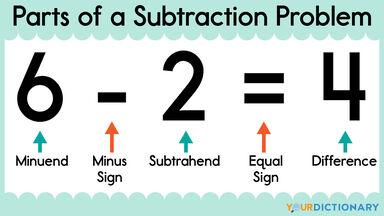# What Are the Main Parts of a Subtraction Problem?

Are you looking for information on the parts of a subtraction problem? Whether you're learning this information for yourself or are looking for ideas on how to explain or teach it to someone else, you've come to the right place.labeled parts of a subtraction problem

## 3 Main Parts of a Subtraction Problem

Every subtraction problem has three main parts: the minuend, the subtrahend and the difference. Learning the parts of a subtraction problem is an important step toward building strong mathematics skills.

### The Minuend

In a subtraction problem, the minuend is defined as the number from which another number is to be subtracted. It always comes before the minus (-) sign.

Tip to Remember - You will minus another number from the minuend.

### The Subtrahend

The subtrahend of a subtraction problem is defined as the number that will be subtracted from another. It is always between the minus (-) sign and the equal (=) symbol.

Tip to Remember - You will subtract the subtrahend from another one.

### The Difference

In math, the difference is defined as the result of subtracting the subtrahend from the minuend. In other words, it is the answer or the solution to a subtraction problem. It always comes after the equal (=) sign.

Tip to Remember - You're done with the problem once you calculate the difference

### Memory Tips for Minuend, Subtrahend and Difference

Need a little help remembering the parts of a subtraction problem? To help you remember the order of the minuend, subtrahend and difference, commit this phrase to memory: "Me first. Subtract me. Done with the problem."

• m (for minuend) - me first, it comes first in the problem
• s (for subtrahend) - subtract me, it comes after the minus sign
• d (for difference) - done with the problem, it is the answer to the problem

Since this phrase follows the order in which each part appears in a subtraction problem, it will help you remember how to label the various parts.

## Example: Identifying the Parts of a Subtraction Sentence

Consider the subtraction sentence 6 - 2 = 4. Each number in this problem represents one of the three main parts.

• minuend - The minuend in the sample problem listed above is 6. It is the number from which the other number (2) will be subtracted.
• subtrahend - The subtrahend in the example problem above is 2. It is the quantity that is being subtracted from the other number (6).
• difference - The difference in the sample problem above is 4. It is the amount left over when the subtrahend is subtracted from the minuend. In other words, it is the answer to the problem.

To help solidify your understanding of these terms, review the example subtraction problem with each part labeled: 6 (minuend) - 2 (subtrahend) - 4 (difference).

## Example: Identifying the Parts of a Subtraction Problem

Subtraction problems aren't always set up as subtraction sentences. Sometimes they are written in a stacked format. In this case:

1. The minuend appears on the top, before the subtrahend.
2. There is a minus sign (-) on the next line in front of the subtrahend.
3. The subtrahend is underlined.
4. The difference appears below the line under the subtrahend.

The subtraction sentence 50 - 40 = 10 would be written as follows using this format:

## Example: Finding the Parts in a Subtraction Word Problem

Now that you can find the parts of a subtraction problem when the subtraction sentence is already written out, try your new skills with a word problem. Consider the following scenario.

Steve and Theresa ordered a pizza. The pizza had 8 pieces. Steve ate four pieces and Theresa ate one. How many pieces of pizza are left?

You'll need to review the word problem and determine each part of the subtraction problem in order to change the word problem to a subtraction sentence that you can solve.

1. Which number will another number be subtracted from? That will be the minuend, which is the 8 pieces that the pizza originally had before any were eaten.
2. Which number will be subtracted from another? That will be the subtrahend, which is the number of pieces that have been eaten. Steve ate 4 pieces and Theresa at 1 piece, so a total of 5 pieces are gone. The subtrahend is 5.
3. The answer to the problem lies in figuring out how many pieces remain. You'll need to calculate the difference, which will require subtracting the subtrahend from the minuend. The difference is 3.

This problem will look like 8 - 5 = 3 when written as a subtraction sentence. The minuend is 8, the subtrahend is 5 and the difference is 3. Using the strategy of breaking word problems down into their three main parts can really help you build strong math skills.